﻿ M.Sc. Operational Research(OR) Coaching Delhi, Mumbai•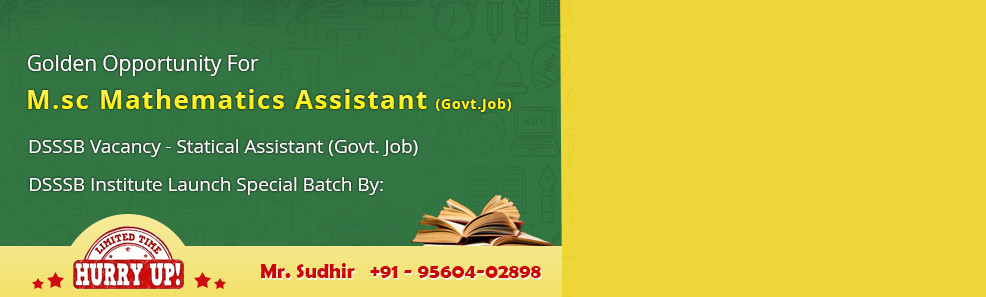•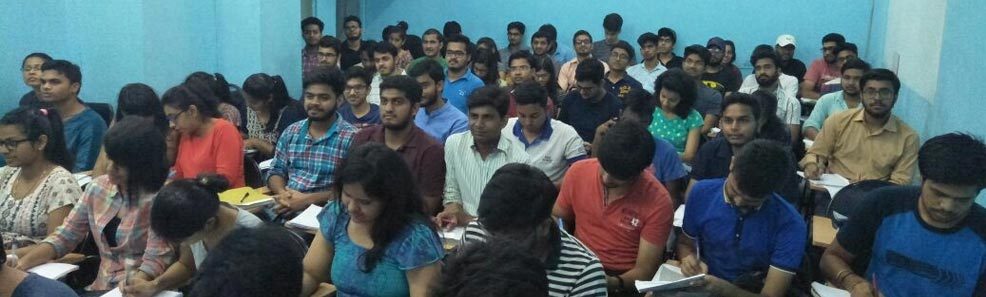•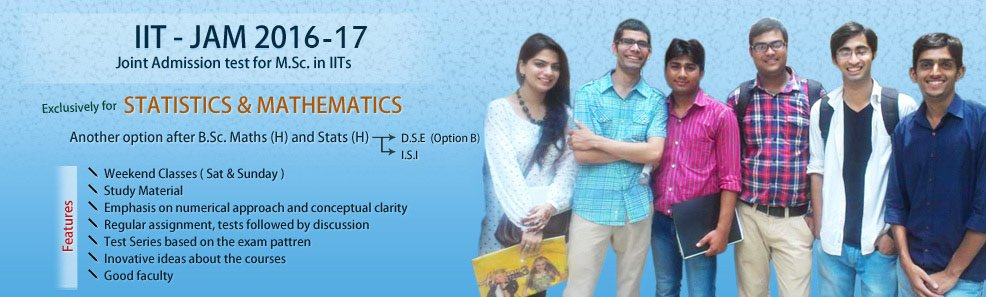•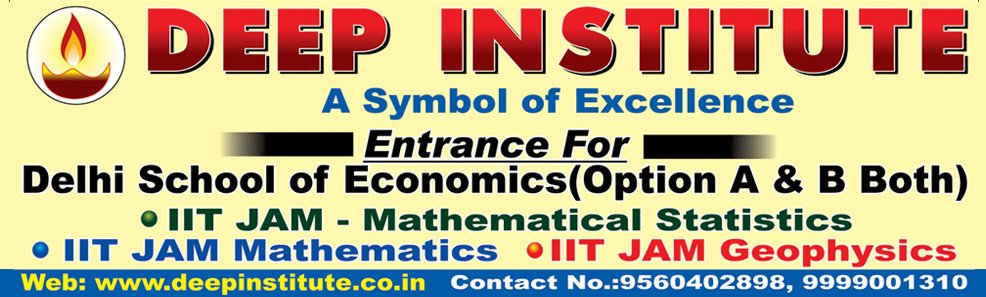•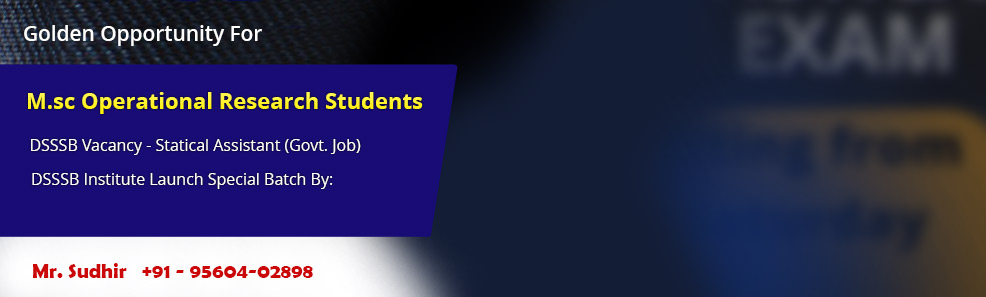•# MSc in Operational Research DU

Operational Research (OR) is the area of study where the students learn to use advanced analytical techniques for better decision making. Sometimes it is also called as Operations Research, Industrial Engineering or Management Science. People having Master's degree (and skills) in OR are perfect for jobs in decision support, marketing analysis, business analytics and logistics planning.

New Delhi based Deep institute offers coaching and tuitions for those wanting to prepare for M.Sc. Operational Research entrance examination conducted University of Delhi (DU).

### Syllabus For M.Sc. Operational Research Entrance Delhi University (DU):

Mathematics :

1. Algebra

• Linear Algebra. Vector space, subspace and its properties, linear independence and dependence of vector, matrices, rank of matrix, reduction to normal forms, linear homogeneous and non-homogeneous equations, Cayley-Hamilton theorem, characteristic roots and vectors.
• Theory of equation. De Moivere's theorem, relation between roots and coefficient of nth degree equation, solution to cubic and biquadratic equation, transformation of equation.

2. Calculus

• Diff. calculus. Limit and continuity, differentiability of functions, successive differentiation, leibnitz's theorem, partial differentiation, Euler's theorem on homogeneous functions, tangents and normals, asymoptotes, singular points, curve tracing.
• Integral calculus. Reduction formulae, integration and properties of definite integrals, quadrature, rectification of curves, volumes and surfaces of solids of revolution.

3. Differential Equation

• Linear, homogeneous equation, first order higher degree equations, algebraic properties of solutions, linear homogeneous equation with constant coefficients, solution of second order differential equation, linear non-homogeneous differential equations.

4. Real Analysis

• Neighbourhood, open and sets, limit point and Bolzano weirstrass theorem, continuous functions, sequences and their properties, limit superior and limit inferior of a sequence, infinite series and their convergence, Rolle's theorem, mean value theorem, Taylor's theorem, Taylor's series, Maclaurin's series, maxima and minima, indeterminate forms.

5. Statistics

• Measures of central tendency and dispersion and their properties, skewness and kurtosis, introduction to probability, theorem of total and compound probability, Bayes theorem, random variables, probability mass and density functions, mathematics expectation, movement generating functions, Bionomial, Poisson, geometric, exponential and normal distribution and their properties, method of least squares, correlation, and introduction to sampling, sampling distributions and tests of significance based on, Chi-square and F-distributions.

6. Operational Research

• Definition & scope of Operational research, formulation of simple linear programming problems, simplex method and basics of duality.
Characteristics of inventory system, simple economic, lot size inventory models, reorder level, simple single period stochastic inventory model.
Definition of queues and their characteristics, Queuingmodels with markovian input and Markovian service, M/M/I & M/M/C queuing models.
Definitions of reliability, Availability, Reliability of multicomponent systems, failure distributions, exponential and Weibull.

7. Computer Science

• Some basic concept

FOR PROPER GUIDANCE
Contact -- 09999001310 (SUDHIR SIR)

DEEP INSTITUTE